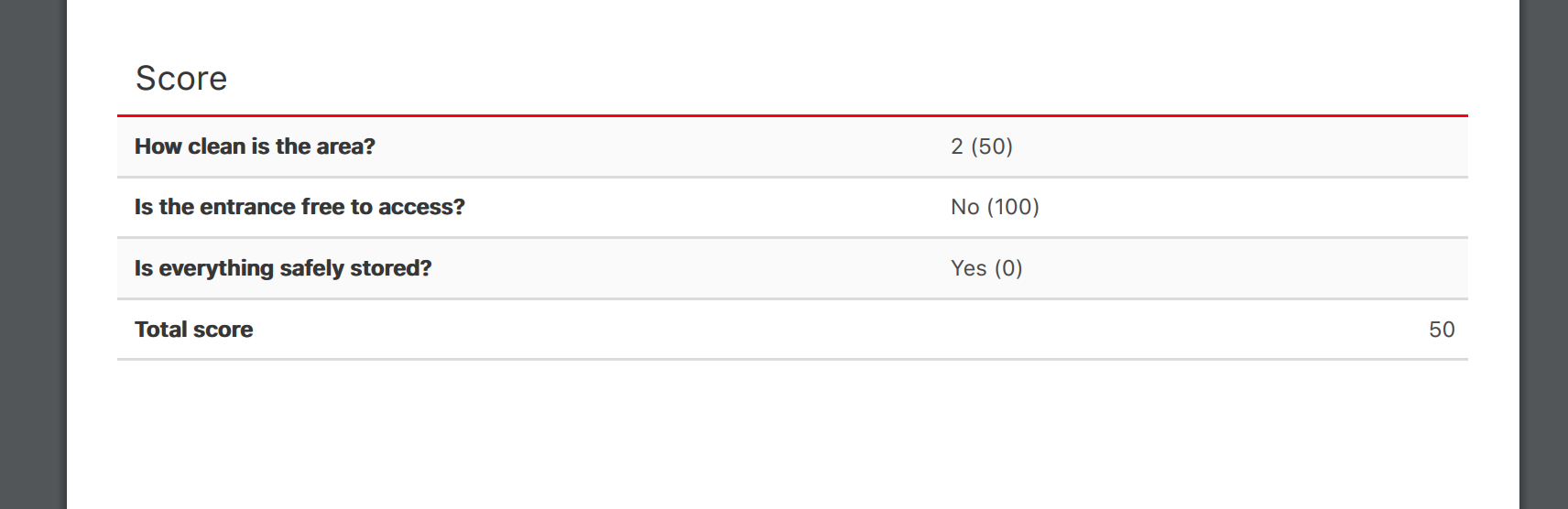Help Center

# Data keys & data values [Premium feature]

## With data values, you can add values or texts on a question level. Two functions are provided: you can make calculations and weigh them. Or you can easily integrate this functionality with one of your systems.

What are data keys?
Data keys are unique codes you use to create formulas, calculate and weigh scores on question level.  Users won't see these values or texts.

EXAMPLE

Every question answered with "Yes" needs to have value: 100. and every question answered with "No" needs to have value: 0.
When all questions are answered you want to calculate the average of all of these values. You do this by creating a formula to calculate the end score.

How do I add a data value on question level?

Go to the template editor and then Step 2: Sections and questions.
Make sure to check the "Enable data keys and values per question" box.

Create a new question, choose the name and type.

For this example, I chose 'Single select' as the answering type. The inspector can rate the cleanliness of the area with 1 to 4. 1 being the lowest and 4 being the highest.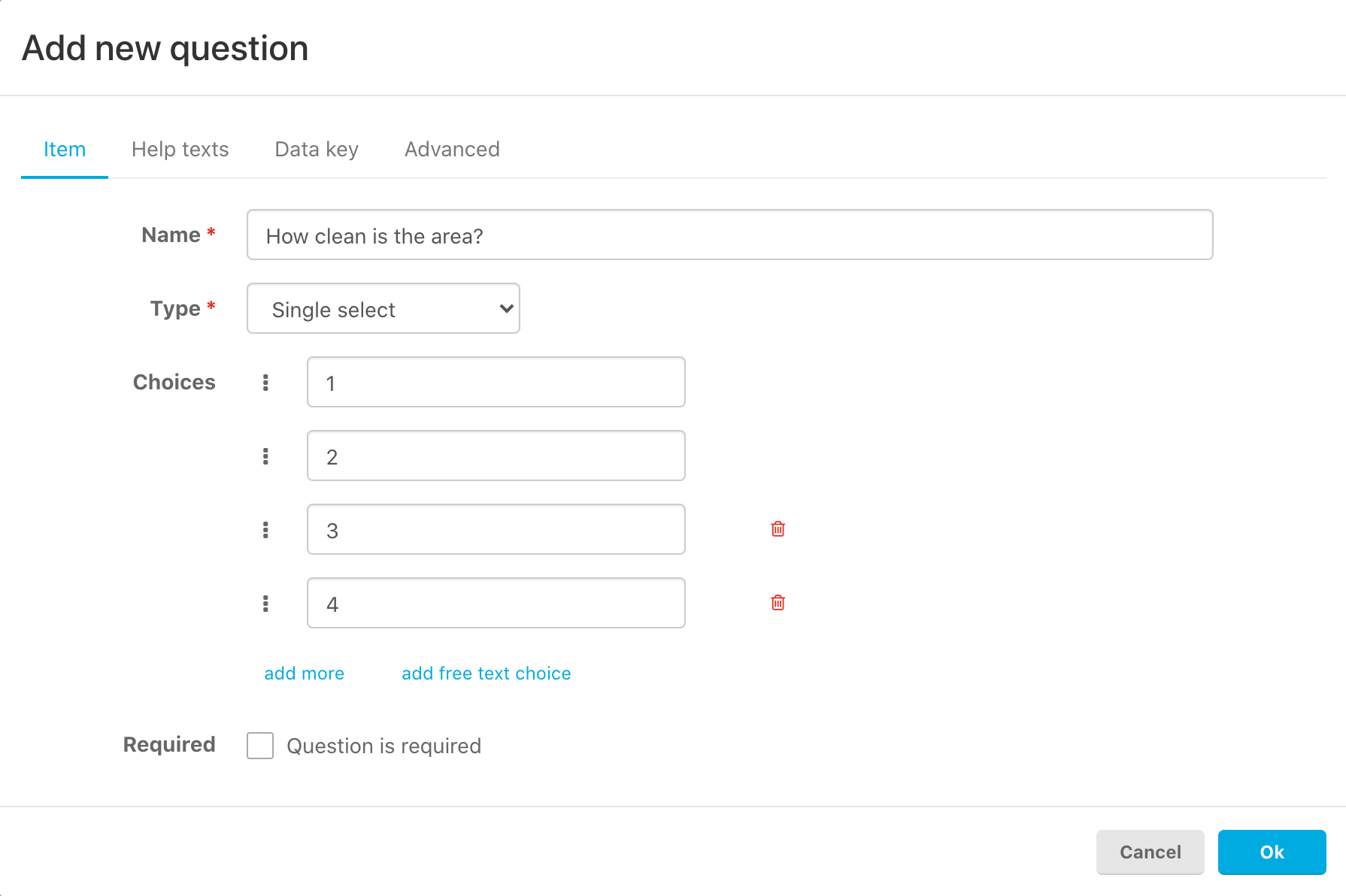Hit the tab 'Data key' and choose for data value type: 'Number'. Change the data key to a single word to make the formula easier. Such as; Item_1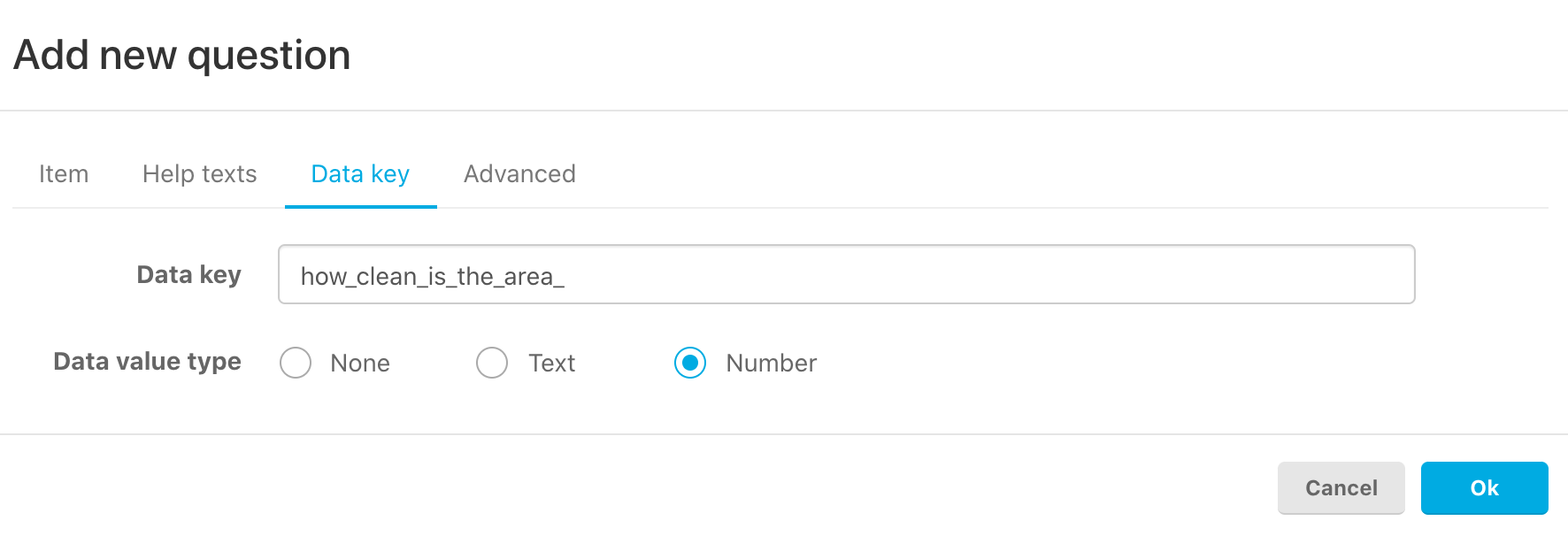Go back to the 'Item' tab and on the right side of every choice, you can give in the value.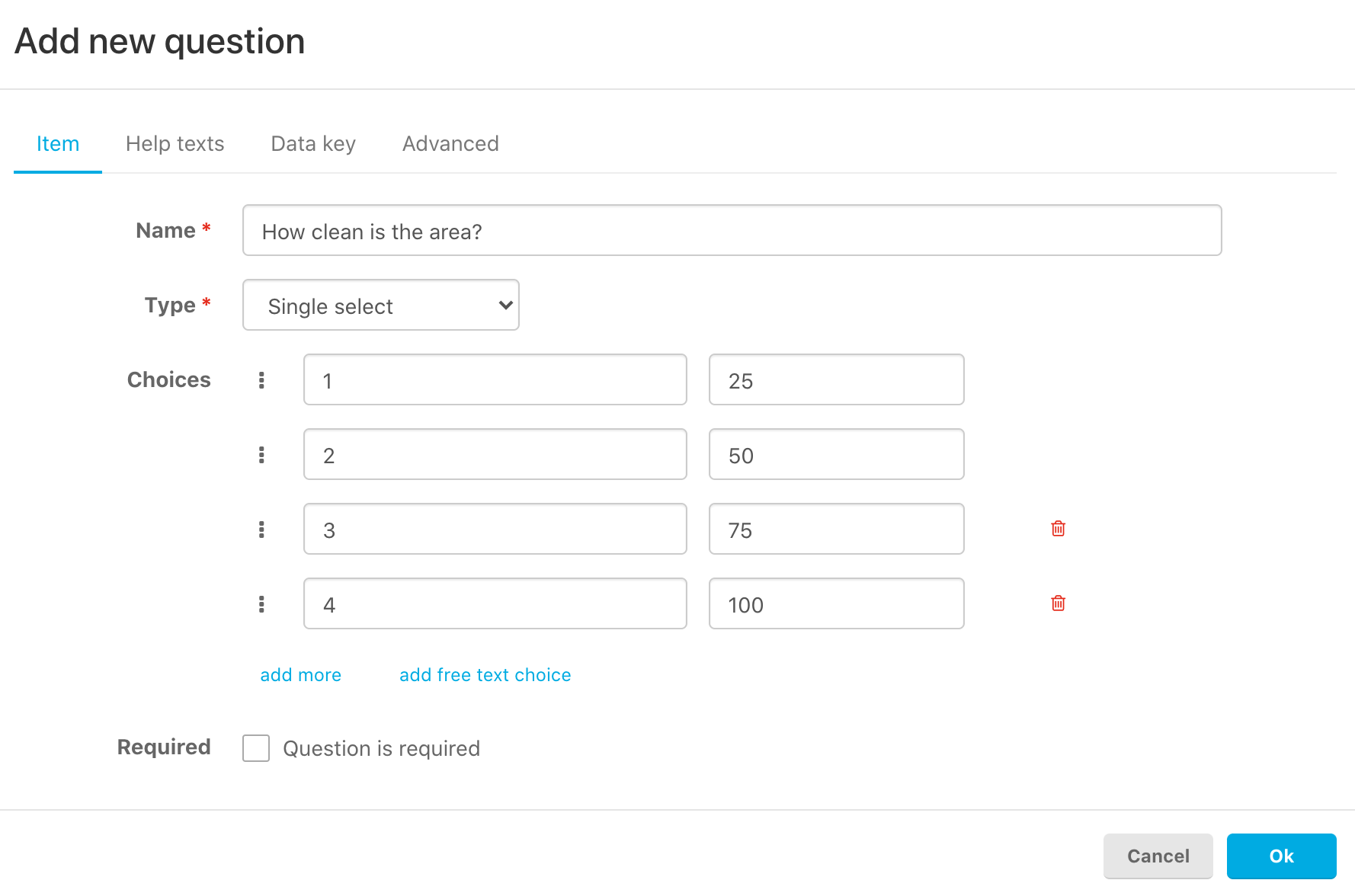Save the question by hitting 'Ok' and create some more questions to be able to create a formula with an end score.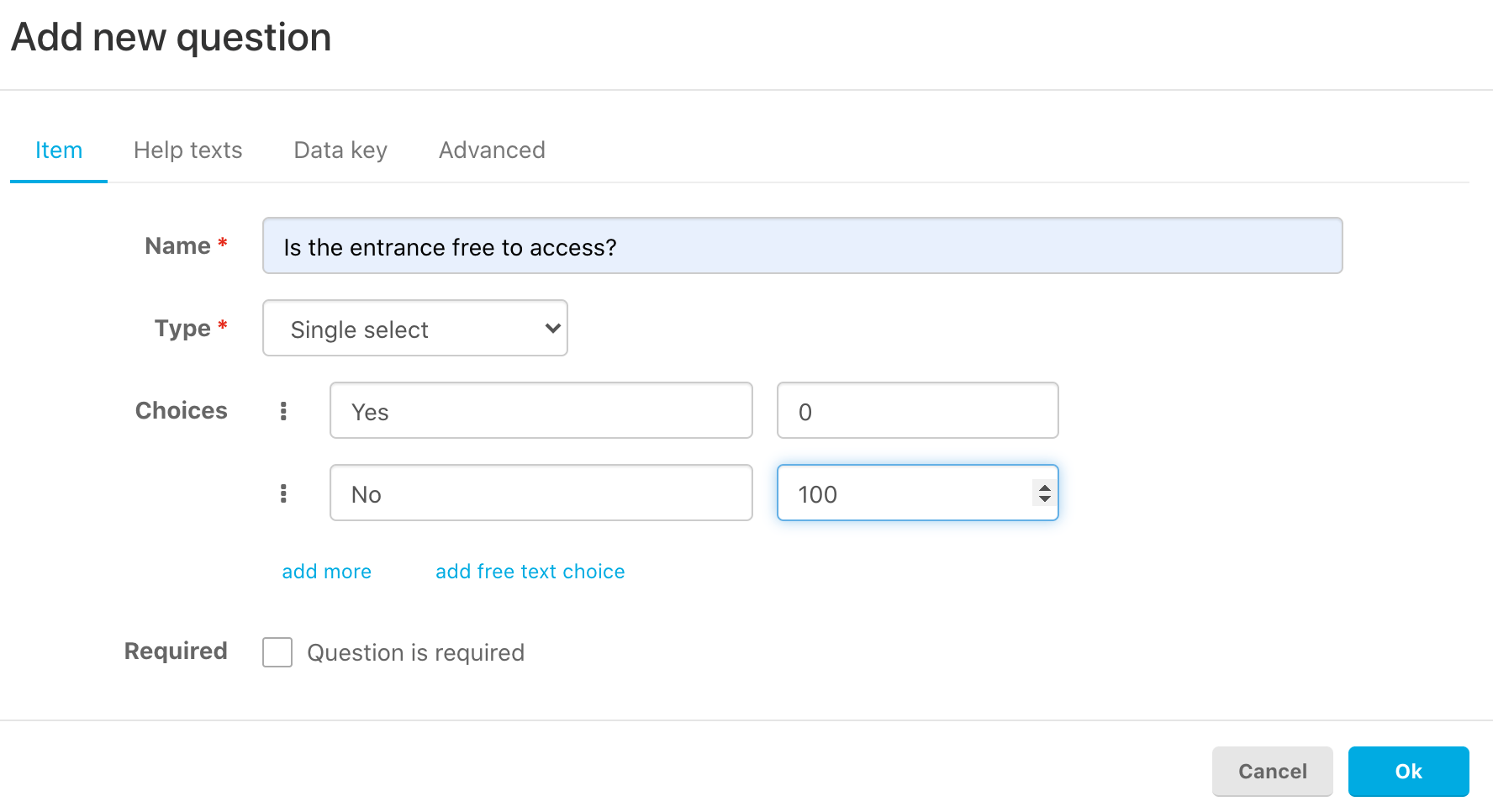When you have created multiple questions, you are ready to create the formula and calculate the end score.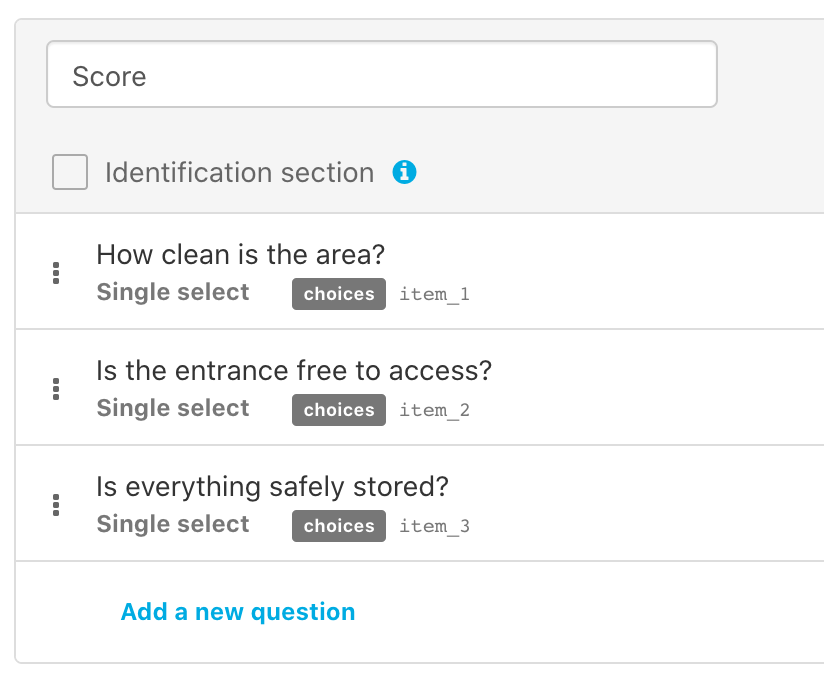How to create the formula that will calculate the end score?

Add a new question with type 'Number' and go to tab 'Advanced'.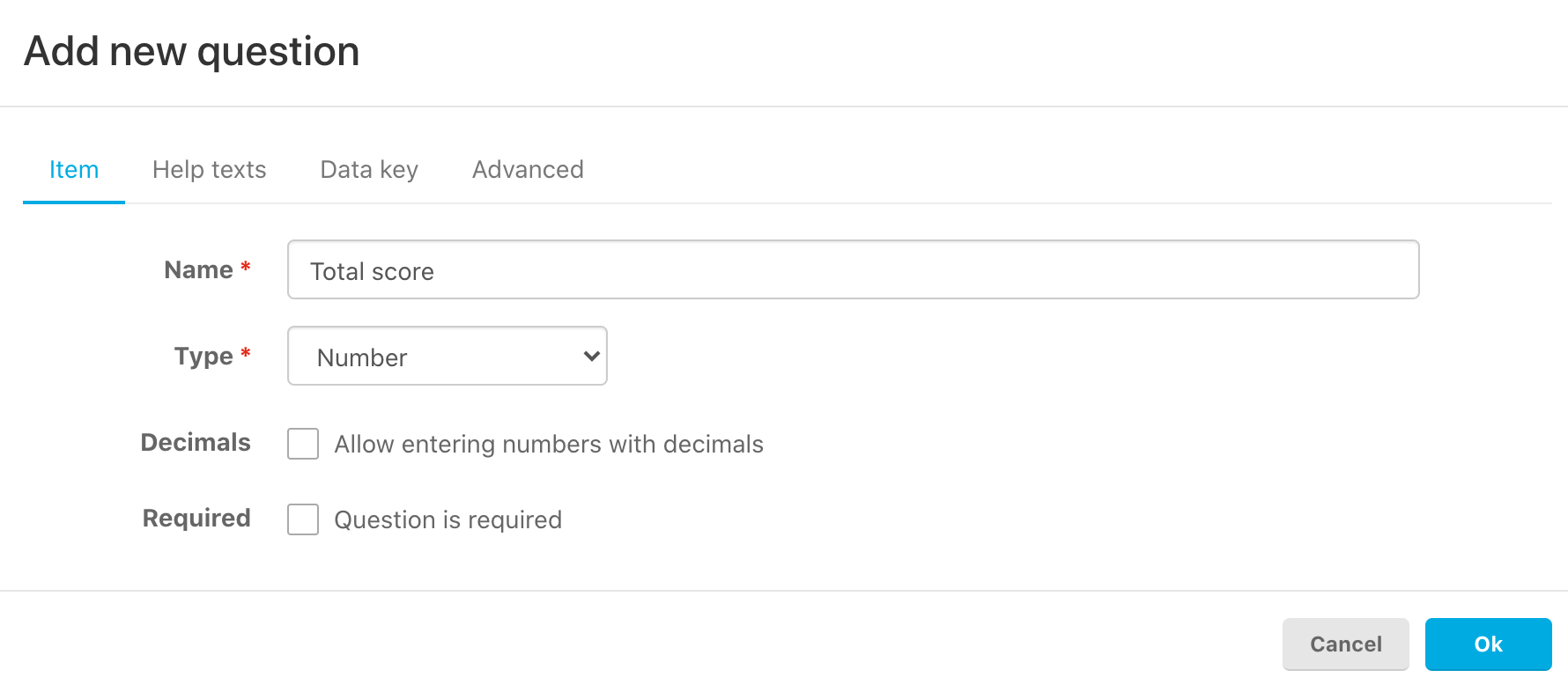Create a formula to calculate an average of all the answers given.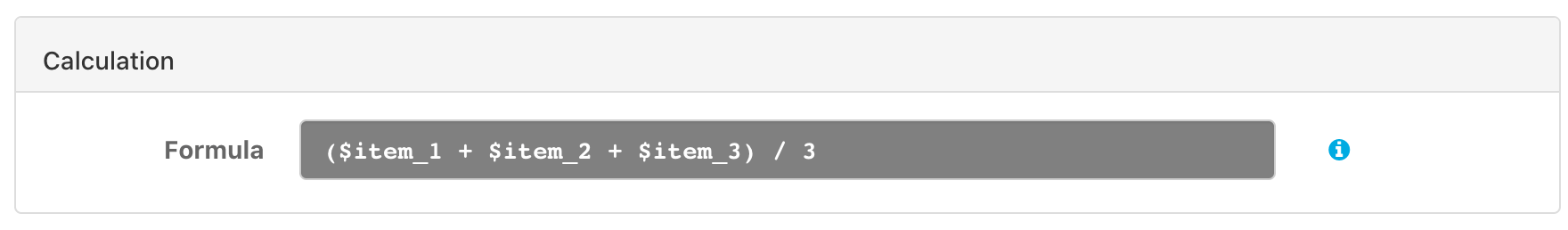This formula calculates the average of all of the three questions. You can choose to show the result in the app for the user to see. Or you can hide the end score in the app, then it will only be shown in the PDF report.

Hit 'Ok' to save the formula.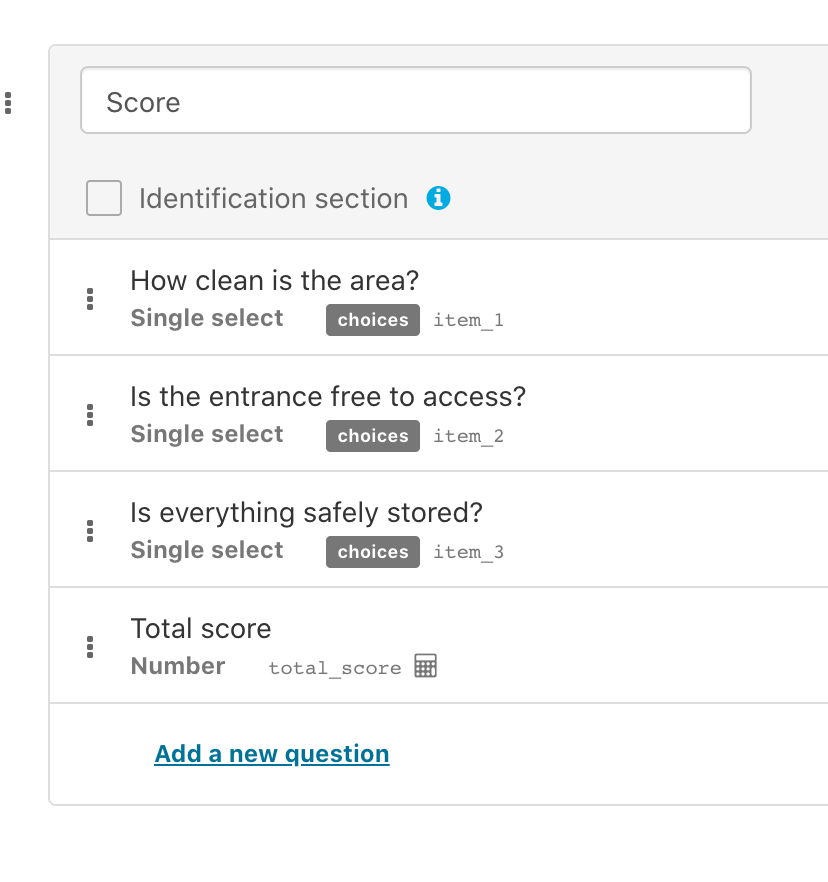Shown in the app: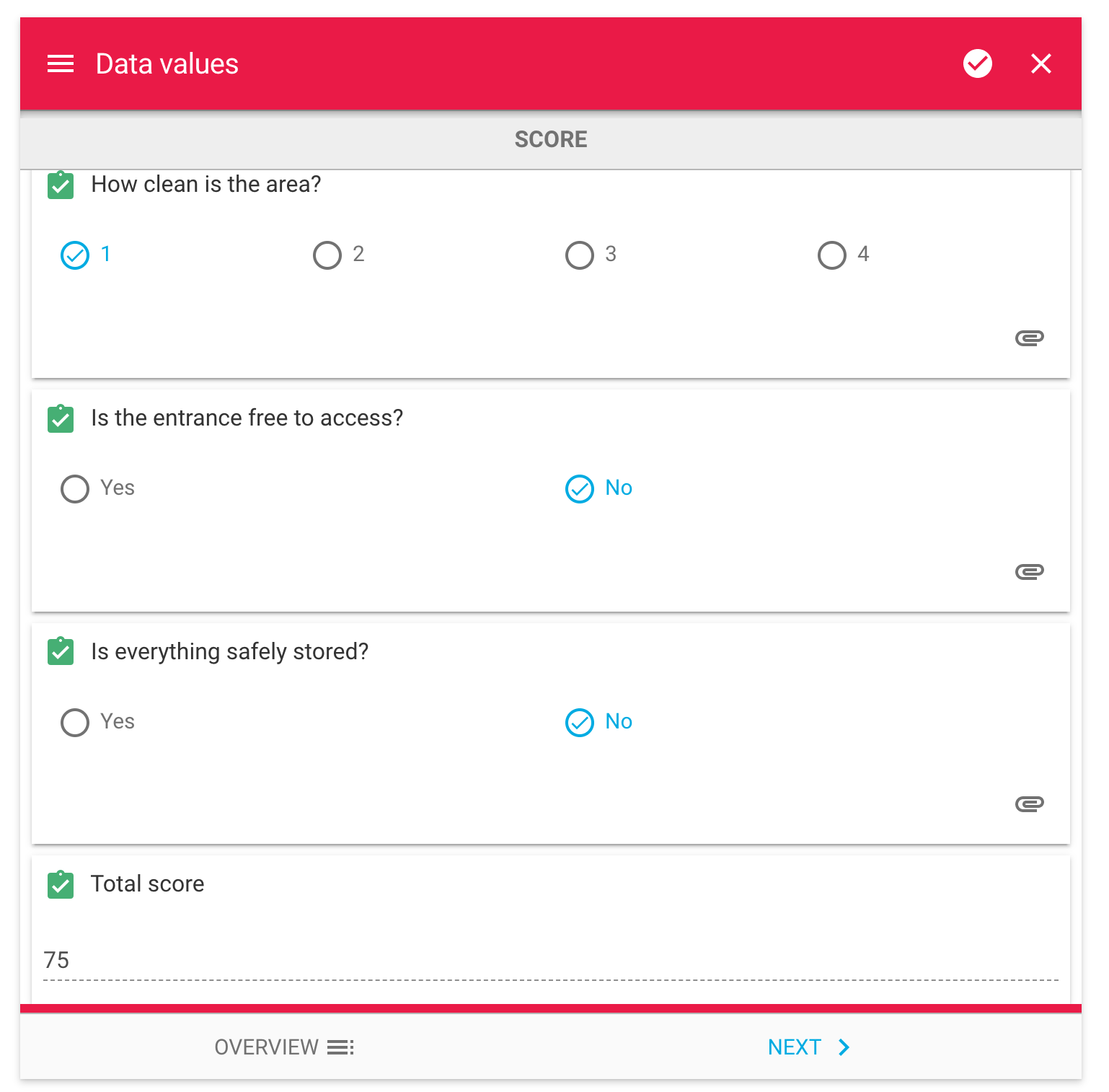Shown in the PDF report: